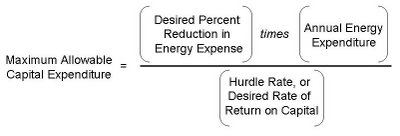## Monday, November 27, 2006

### Capital “Secrets” of Energy Cost Control

Sooner or later, an organization that is serious about energy cost-control will pursue capital projects. By that, we mean investments in high-cost equipment that will be financed over a number of years. These investments include key energy-using hardware such as boilers, air compressors, chillers, metering systems, etc.

You will recall an earlier post that discussed the merits of “projects” versus “processes” as energy cost-control strategies. When seeking results strictly through capital projects, here’s the basic financial equation for project evaluation:This is derived from the capitalized income concept, which divides net income by a discount (hurdle) rate to yield a capitalized value. You’ll see that formula in real estate, for example, for determining a property’s capitalized value. In this instance, however, we are capitalizing an increment of net income—expressed here as a percent reduction in energy expenses. We can use “income” interchangeably with “expense” because a dollar saved is a dollar earned.

When using this formula as written, one calculates the maximum capital to be expended, given (1) a current level of energy expenditure, (2) a hurdle rate, and (3) a desired percent reduction in energy expenses. An alternative approach for the same formula is to insert a fixed amount for capital expenditure and solve instead for the desired percent reduction in energy expense. In other words, this second approach describes the percent savings that should be achieved by a certain amount of capital expenditure. Notice also how the volume of allowable capital expenditure decreases as risk rises. The higher the risk, the higher the discount rate—the denominator of the equation shown above (rate of return on capital).

And now, the secrets of capital investment….

Remember that capital projects do not always guarantee results. To offset the risk of failure, finance people will arbitrarily raise the discount rate for evaluating energy projects. Just how much of an adjustment is needed is a matter of individual judgment. A higher discount rate yields less capital for an energy manager to work with. The energy manager can and should offset capital investment risk, effectively lowering the discount rate. How? New capital assets should be accompanied by behavioral and procedural changes that reduce energy waste. Staff need to be aware of wasteful practices. Energy-smart behavior needs to become a part of standard operating procedures. Energy cost-control performance needs to be wrapped into daily job accountabilities. This effort requires some communication, discipline, and cross-departmental coordination. Companies that develop these management skills can effectively reduce the risk on their future capital projects.

Labels: